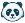# [New Quizzes] New Quizzes: Multiple Answers in a Formula Question

This idea is based on extending the Formula Question type to allow for multiple answers to be entered by students.

Currently, the Formula question type is fine for simple calculated answer questions that only ask for one final response from students. We have several instructors that would like to generate data based on ranges, but also to ask for several answers based on that generated data.

One example of this would be a financial statement or budget sheet. Instructors and Designers would like to generate random data elements for some parts of these sheets, but ask for multiple answers from the same set of generated data such as the following list of questions:

"What is the Inventory Turnover? What is the AR Turnover? What is the AP Turnover? What is the Inventory Period? What is the AR Period? What is the Operating Cycle? What is the Cash Cycle? What is Net Working Capital for 2013?"

Each answer is based on the same data, but uses different formulas to determine the correct result.Community Member

"Open for conversation since 2015," yea. We are still talking about it but nothing is being done.Community Explorer

I want to add my voice in strong support of this, with an additional suggestion (in case it isn't already mentioned in the myriad of supporting comments) :

Let us assign the intermediate answers to a variable of their own. This would allow us to give partial credit to students who got part of a question right, even if the mistake happens in one of the first answers. Something like the following:

"A random variable X returns values 1,3 or 10. The probability of X=1 is [p1], and the probability of X=3 is 0.1 higher.

What is the probability of X=3? [[p3]]

What is the expected value of X? [[E]]"

The first question would receive credit if the answer equals p1+0.1, but whatever the answer is, that value is assigned to p3.

The second question would receive credit if the answer equals p1*1+p3*3+(1-p1-p3)*10. That way, students can get full credit on the second part even if they missed the first part.

This would be particularly powerful in combination with "score by multiple formulas" as a previous commenter has suggested. This would allow an instructor to assign credit for the second question if either E=p1*1+p3*3+(1-p1-p3)*10 (i.e. working with whatever the student reported earlier) or E=p1*1+(p1+0.1)*3+(1-2p1-0.1)*10 (i.e. working out the expectation without relying on the intermediate answer).

I realize there's a tradeoff between simplicity of use and versatility. But the following seems convenient: You allow teachers to assign a variable to the student's entries (e.g. by indicating response fields with [[ ]] brackets). And then you allow us to determine scoring rules based on formulas that can call on all the variables (user-entered or pre-populated).

In this case, I could say that p3=p1+0.1 is worth 0.2 points, and E=p1*1+p3*3+(1-p1-p3)*10 is worth 0.8 points.

If I want to be fancy, I could allow the two different options for E by writing OR(E=p1*1+p3*3+(1-p1-p3)*10,E=p1*1+(p1+0.1)*3+(1-2p1-0.1)*10).Community Member

This is an important feature for science and math classes. It needs to be added to the question types.Community Participant

Why on earth are our pledges so brutally ignored by Canvas"technical support"?. We are FORCED to use Canvas at my institution. It's obvious that selling and marketing an LMS has nothing to do with the final users 😞Community Member

This is extremely frustrating that Canvas management don't even care to respond to our requests. There are several other idea conversations requesting this same feature.Community Member

This is a key feature that is needed!Community Team
Status changed to: NewCommunity Team
Status changed to: NewCommunity Team
Status changed to: Added to Theme